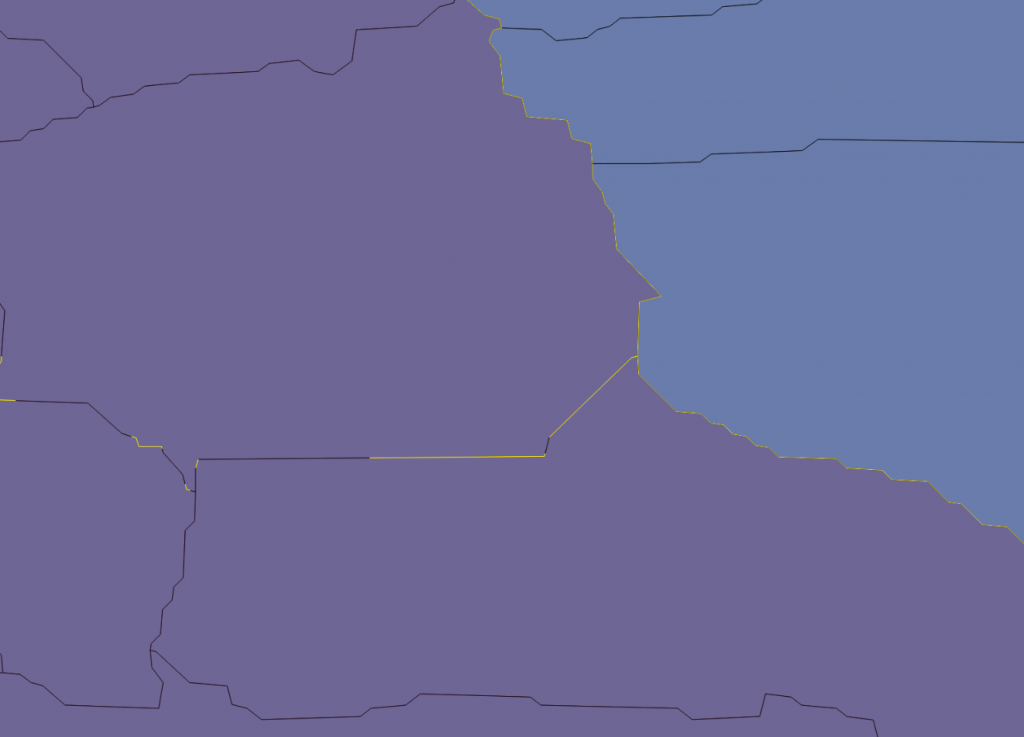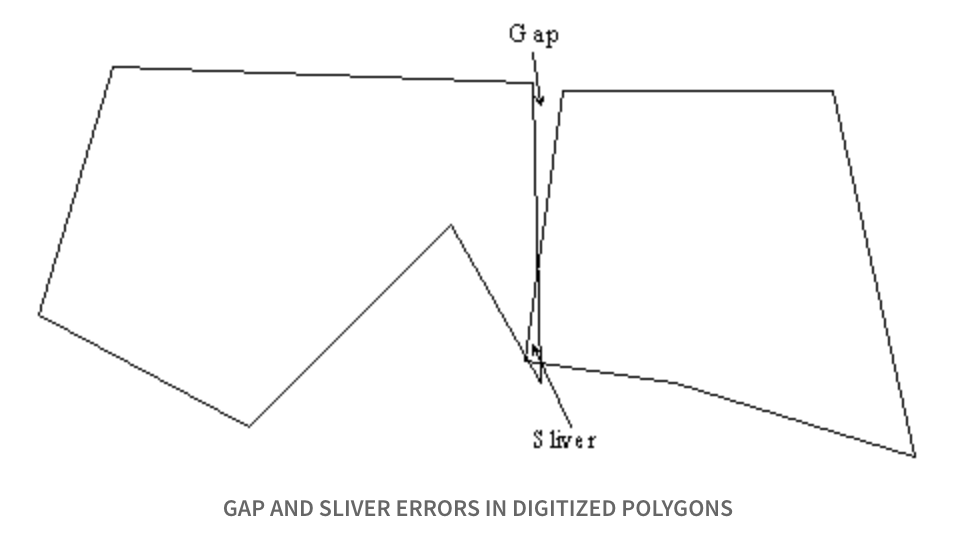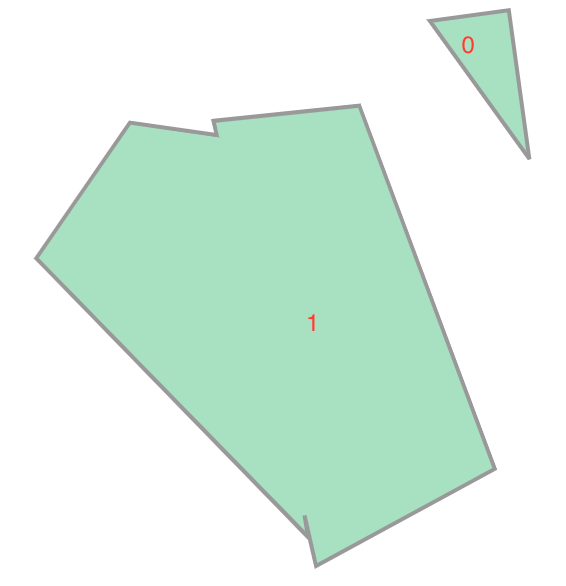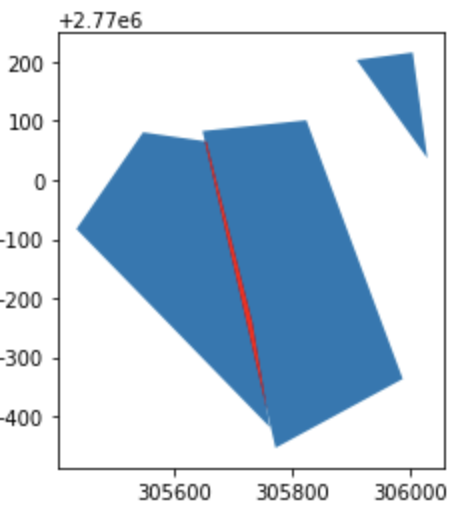#2019 iT 邦幫忙鐵人賽DAY 23
0
AI & Data

## Day23 資料品質：幾何檢查(續篇)

• gap and sliver polygon
• 檢查方法
• 修正

## gap and sliver polygon## 檢查方法A valid MultiPolygon may not collect any overlapping polygons. --- Shapely

``````import geopandas as gpd
from shapely.geometry import MultiPolygon, Polygon
````````````from shapely.geometry import MultiPolygon, Polygon
data=[]
for i in range(len(test.geometry)):
data.append(test['geometry'][i])
mp = MultiPolygon(data)
mp_obj = gpd.GeoSeries(mp)
mp_obj.is_valid
``````

## 修正

``````union = test.geometry.unary_union
union
``````1號polygon是被合併的部分，我們試著找出邊界不一致的區塊

``````# gap
gap = list(union)  #
gap=gap.difference(test['geometry']).difference(test['geometry'])

````````````# sliver
sliver=test['geometry'].intersection(test['geometry'])
````````````ax=mp_obj.plot()
gap_obj = gpd.GeoSeries(gap)
sliver_obj = gpd.GeoSeries(sliver)
ax=gap_obj.plot(ax=ax,color='yellow')
sliver_obj.plot(ax=ax,color='red')
``````(工人智慧?XD)